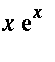Introduction to the Mgfun Package

The Mgfun package consists of procedures to compute with special functions and combinatorial sequences that are implicitly defined as solutions of systems of linear difference-differential equations. Its syntax is very close to that of the gfun package. In the same spirit as with the latter, a typical use of Mgfun to compute a sum or integral is the following. First one obtains systems that define the summand or integrand by calls to dfinite_expr_to_sys or by combining known systems by using `sys+sys` and `sys*sys` . Then one derives the sum or integral by a call to sum_of_sys or int_of_sys . Finally one has to solve the system to obtain a closed form (if one exists).

> with(Mgfun);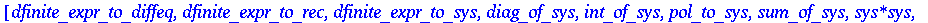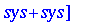In the sequel, we exemplify the use of the package by working out the evaluation of a sum and of two integrals.

Closed Form Evaluation of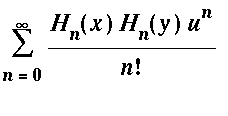Closed Form Evaluation of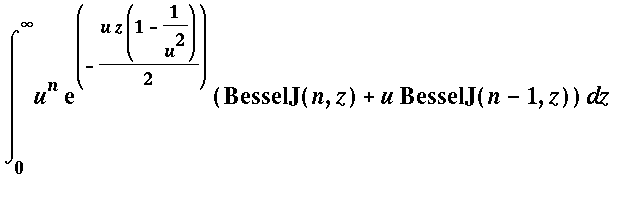Chebyshev Coefficients of